Next: 5.8 Mixed-Mode Up: 5. Contacts and Boundaries Previous: 5.6 Contact Voltage Variable

# 5.7 Example

As an example a one-dimensional semiconductor structure with a simple ohmic boundary condition is considered. The index of the quantities is the number of the grid point they belong to, with 0 being the left contact. For the electron concentration n0 = N0 with N0 being a constant value depending only on the doping of the semiconductor. To further simplify the example only the static Poisson equation and the static continuity equation for electrons are considered. Furthermore, the separate variable for the electron contact current ICn is omitted. For a one-dimensional device the segment constitutive relations read

 fS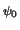=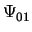+ q . n0 . V0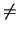0 fS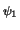=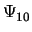+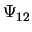+ q . n1 . V1 = 0fSn0 = I010 fSn1 = I10 + I12 = 0with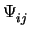=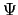(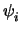,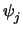) = -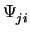Iij = I(ni, nj,,) = - Iji

At the boundary, the constitutive relations are

 f=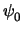-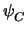= 0 fn0 = n0 - N0 = 0 fIC = IC + fSn0 = 0 fQC = QC + fS= 0

The boundary constitutive relations will be used to determine the quantity values at the boundary while the segment constitutive relations will be used to build up an expression for the boundary charge QC and for the boundary current IC. This is achieved by the boundary models which set the appropriate entries in the transformation matrix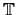B which reads

 tx, y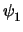n0 n1 IC QC1 n0 n1 1 IC 1 QC 1

The solution vector x contains the following quantities

 x =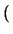,, ... n0, n1, ..., IC, QC, ...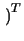For voltage controlled contacts with V0 applied to the contact one gets

 f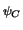=- V0 = 0

When applying the current I0 to the contact fchanges to

 f= IC - I0 = 0

The system matrix for iteration step k is

 jx, yn0IC QC r-1 1 fk n0 -1 fn0k-1 fk IC --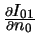-1 fICk QC -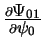-q . V0 -1 fQCk

As the constitutive relations for the quantities, n0, and IC are eliminated first, one ends up with the following matrix

 jx, yr-fk - fICk + fk .+ fn0k .Next: 5.8 Mixed-Mode Up: 5. Contacts and Boundaries Previous: 5.6 Contact Voltage Variable
Tibor Grasser
1999-05-31